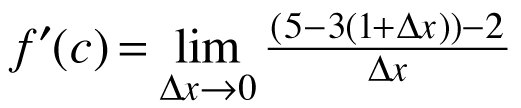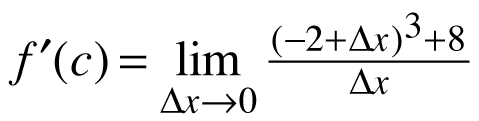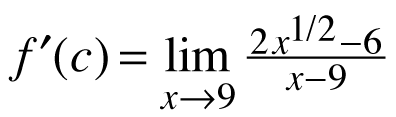### Home > CALC > Chapter 5 > Lesson 5.2.4 > Problem5-99

5-99.
1. For the following functions, find f(x), c, f ′(x), and f ′(c). Homework Help ✎

1.2.3.This is Hana's Definition of the Derivative. Notice that Δx is equivalent to h.

f(x) = 5 − 3x where c = 1
so f '(x) = −3 and f '(1) = −3See hint in part (a).This is Ana's Definition of the Derivative.